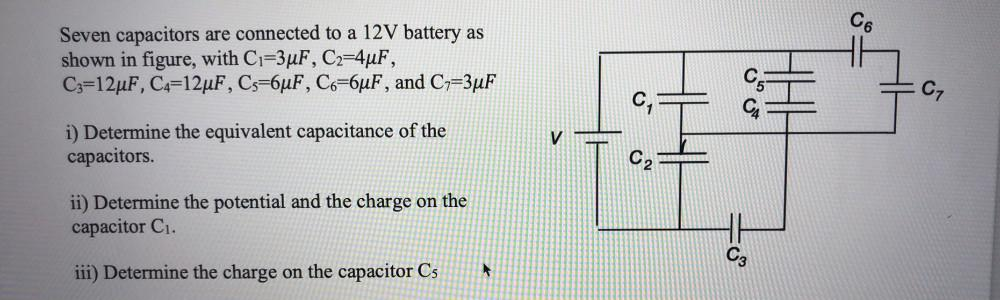Question:

# Co Seven capacitors are connected to a 12V battery as shown in figure, with C1=3uF, C2=4uF, C3=12uF, C4=12uF, Cs=6uF, Co=6uF, anCo Seven capacitors are connected to a 12V battery as shown in figure, with C1=3uF, C2=4uF, C3=12uF, C4=12uF, Cs=6uF, Co=6uF, and C7=3uF 05 CZ C CA V i) Determine the equivalent capacitance of the capacitors. C ii) Determine the potential and the charge on the capacitor C1. C3 iii) Determine the charge on the capacitor Cs